# Basic Geometry: Circles Chapter Exam

Exam Instructions:

Choose your answers to the questions and click 'Next' to see the next set of questions. You can skip questions if you would like and come back to them later with the yellow "Go To First Skipped Question" button. When you have completed the practice exam, a green submit button will appear. Click it to see your results. Good luck!

### Page 1

#### Question 1 1. The red segment pictured on the circle represents: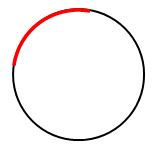#### Question 2 2. If the area of the square is 64, what is the area of the circle, rounded to the nearest whole number?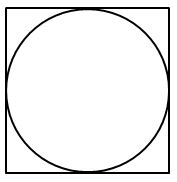#### Question 4 4. What is the area of the shaded area, rounded to the nearest whole number?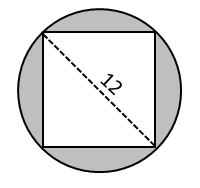### Page 2

#### Question 8 8. In the pictured circle, the measure of angle ACB is 22 degrees and the measure of angle BXD is 120 degrees. What is the measure of angle CAD?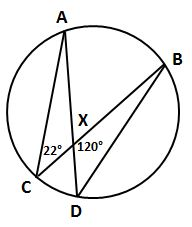#### Question 9 9. If angle AOB is 40 degrees, what is the measure of angle ACB?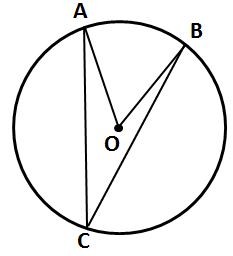### Page 3

#### Question 11 11. What is the line, as shown in the picture, called?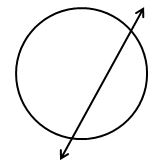### Page 4

#### Question 17 17. If angle ACB is 47 degrees, what is the measure of angle AOB?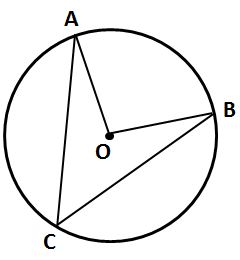#### Question 18 18. What is the area of the shaded area, rounded to the nearest whole number?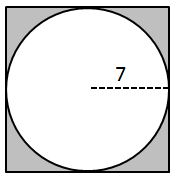#### Question 19 19. If angle OAB is 45 degrees, what is the measure of angle AOB?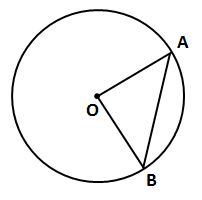#### Question 20 20. If angle ACB is 34 degrees, what is the measure of angle ADB?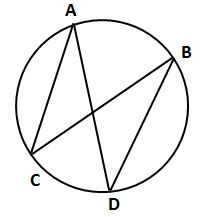#### Basic Geometry: Circles Chapter Exam Instructions

Choose your answers to the questions and click 'Next' to see the next set of questions. You can skip questions if you would like and come back to them later with the yellow "Go To First Skipped Question" button. When you have completed the practice exam, a green submit button will appear. Click it to see your results. Good luck!

Support